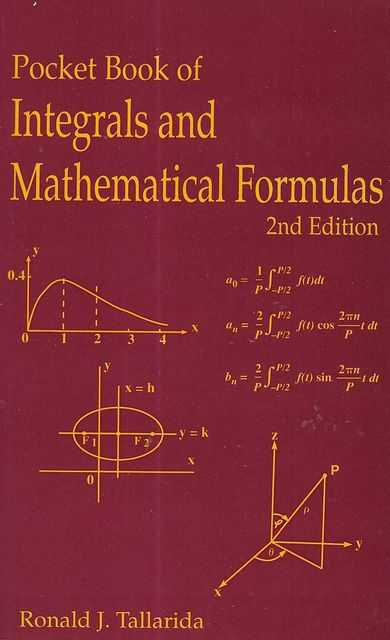#### Author:Ronald J. Tallarida

Sort

Showing 1-1 of 1#### Pocket Book of Integrals and Mathematical Formulas

Price: \$8.00

Publisher: USA, CRC Press: 1992

Seller ID: 249105

ISBN: 0849301424

Condition: Near Fine Condition

Pocket Book of Integrals and Mathematical Formulas, Second Edition, is a handy, compact reference work containing concise discussions of mathematical concepts and formulas frequently needed by students and professionals in engineering, science, applied math and statistics. One of the book's special features is its comprehensive table of integrals arranged and formatted to facilitate the rapid location of the right form. Tables of derivatives; calculus applications; graphs of important Cartesian and polar curves; and formulas from algebra, geometry, trigonometry, calculus and statistics have al... View more info